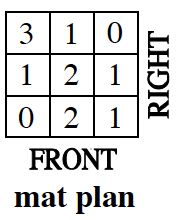### Home > CCG > Chapter 12 > Lesson 12.2.4 > Problem12-104

12-104.Examine the mat plan of a three-dimensional solid at right.

1. On your paper, draw the front, right, and top views of this solid.

2. Find the volume of the solid.

Find the volume of the solid by finding the sum of all the numbers on the mat plan (i.e., the total number of unit cubes used to construct the solid).

$11$ cubic units.

3. If each edge of the solid is multiplied by $5$, what will the new volume be? Show how you got your answer.

The volume of the new solid will be $5^3 = 125$ times the volume of the original.

$(11)(5^3) = 1375$ cubic units.

Use the eTool below to build the 3D figure.
Click on the link at right for the full eTool version: 12-104 HW eTool